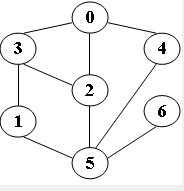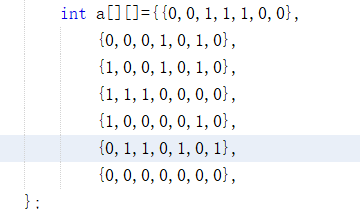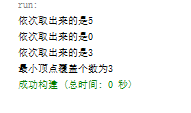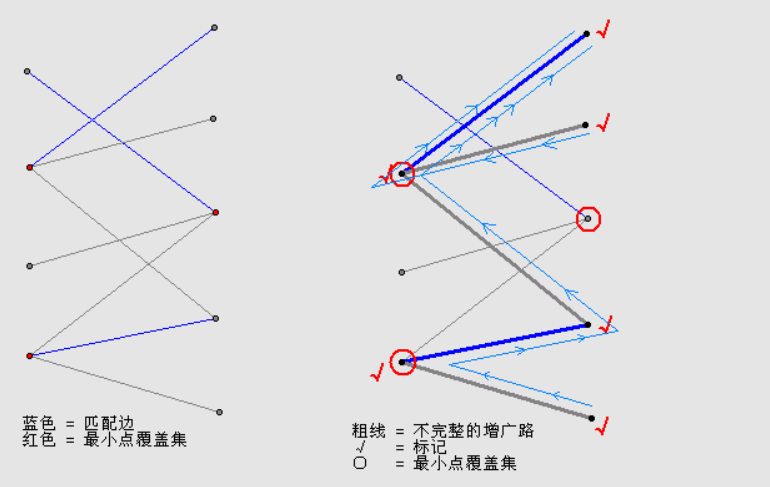•研究论文
• 设计一个求解最小顶点覆盖的分支限界算法，并分析算法的时间复杂度。（描述算法策略并写出代码） 算法策略： 假设G=,V为G的顶点集合，E为G的边集合 算法策略：可以采用贪心加减枝的策略。令一个全局变量bestw为当前...

已知 G 为一个无向图。给定一个 G 的一个顶点子集 U，当且仅当G 中的每一条边必有一个顶点属于 U 或者两个顶点都属于 U 时，称 U 是 G 的一个顶点覆盖（vertex cover），U 中顶点的数量是覆盖的大小(size)。设计一个求解最小顶点覆盖的分支限界算法，并分析算法的时间复杂度。（描述算法策略并写出代码）

算法策略：

假设G=<V,E>,V为G的顶点集合，E为G的边集合

算法策略：可以采用贪心加减枝的策略。令一个全局变量bestw为当前能找到的最小覆盖的顶点数（初始为G中顶点数，表示未找到）。令树中的结点为一个状态，这个状态的信息包括了已选择了的顶点集合A和它的数量num_of_A。还未覆盖的边集合C以及边数num_of_C，接下来可以选择的图中的顶点集合X，以及这些顶点能与C建立联系的边数优先队列Q（意思就是这个顶点能与C中x个边联系，则这个顶点在队列的值就是x；然后优先队列意思就是那个顶点能与C建立联系的边数大，哪个就在队列的前面）。

从一个树种结点扩展左右子树时，我们这样定义向左扩展意为从Q中弹出一个顶点（根据先前优先队列定义，这个顶点能与C中建立最多的联系），然后这个顶点加入到A中，num_of_A++。并且C也要减去与这个顶点有联系的结点，num_of_C也做出相应的调整。X的变化是减去弹出的这个顶点。Q的变化即为弹出。然后将这些信息构成的状态结点添加到左子树上，并且这个结点入队（入的队后面说）。然后检查一下num_of_C，若为0说明找到了一个覆盖集合，然后这个结点不入队.若num_of_A小于bestw，则更新bestw，并且另一个选择序列B=A，以便输出。

向右扩展也一样，从Q中弹出一个顶点，但是这个顶点不加入A中，那么A不变，C不变，X要减去弹出的这个顶点。Q的变化即为弹出。而且向右不可能找到覆盖集合。然后将这些信息构成的状态结点添加到右子树上，并且这个结点入队。

那么再定义一下扩展顺序，所有树中的结点都根据num_of_C来排列，优先扩展num_of_C最小的。也就是维护一个num_of_C的优先队列。若队列空了那么bestw则为对应的顶点数

再来确定一下限界策略，向左扩展前，检查一下num_of_A，若num_of_A+1后大于bestw，则停止扩展左子树，即不入队。去扩展右子树。向右扩展不做这样的检查。

而向左向右扩展都有不能完全覆盖的危险。检查Q,若Q中前bestw的结点的和小于num_of_C或者X的数目小于bestw，则停止扩展。

算法顺序是这样的：

1.初始化根节点加入优先队列中

2.若队列不为空，则从优先队列中取出一个结点进入步骤3，否则退出，输出B

3.扩展这个结点的左右子树，分别对左右子树运用限界，不满足限界则不加入优先队列。满足则加入优先队列

然后这个树的根节点就是未选择任何一个顶点的状态。那么C=V,Q=V。然后再进行上述循环即可

#include <iostream>
#include<queue>
using namespace std;

#define num_of_point 7

int bestw = num_of_point;
int B;
int G;//编号从0开始,无向图说明对称,对角线规定为0
typedef struct pot {
int num;//顶点编号
int a;//可与C建立联系数
pot(int x = 0, int y = 0) {
num = x;
a = y;
}

}pot;

//int num_of_point;

bool operator<(pot a, pot b)
{
return a.a < b.a;//最大值优先
}

typedef struct node {
int matrix[num_of_point][num_of_point];//表示未建立联系边的矩阵
int num_of_c;//未建立联系的边数
int A[num_of_point];//已选顶点的编号,-1表示未选
priority_queue<pot> q;//可选顶点的集合

}node;//树中结点结构体

void init(node* child, node* parent) {

//child->matrix = new int* [num_of_point];
//child->A = new int[num_of_point];
//for (int i = 0; i < num_of_point; i++)
//	child->matrix[i] = new int[num_of_point];

for (int i = 0; i < num_of_point; i++) {
child->A[i] = parent->A[i];
}
for (int i = 0; i < num_of_point; i++) {
for (int j = 0; j < num_of_point; j++) {
child->matrix[i][j] = parent->matrix[i][j];
}
}
child->q = parent->q;
child->num_of_c = parent->num_of_c;
}//拷贝构造函数

bool operator<(node a, node b)
{
return a.num_of_c > b.num_of_c;//最小值优先
}

priority_queue<node> Qoftree;//他就是树

int k = 0;
pot x = p->q.top();
p->q.pop();//弹出一个顶点
for (k = 0; p->A[k] != -1; k++);//找到第一个是-1，表示第一个未做选择的位置以便于插入
p->A[k++] = x.num;//k即为numofA
if (k > bestw)return;//限界策略
for (int i = 0; i < num_of_point; i++)
{
p->matrix[i][x.num] = 0;
p->matrix[x.num][i] = 0;
}//更新一下啊q
pot y[num_of_point];
int kk = 0;
while (!p->q.empty())
{
y[kk] = p->q.top();
p->q.pop();
y[kk].a = 0;
for (int j = 0; j < num_of_point; j++)
{
if (p->matrix[y[kk].num][j] == 1)
y[kk].a++;
}
kk++;
}
for (int i = 0; i < kk; i++)
{
p->q.push(y[i]);
}

p->num_of_c = 0;
for (int i = 1; i < num_of_point; i++) {
for (int j = 0; j < i; j++) {
if (p->matrix[i][j] == 1)p->num_of_c++;
}
}
if (p->num_of_c == 0 && k < bestw) {
bestw = k;
for (int i = 0; i < k; i++)B[i] = p->A[i];
}
if (p->num_of_c != 0 && !(p->q.empty())) {
Qoftree.push(*p);//入队
}
}

p->q.pop();//弹出之后检查，是否还能形成覆盖
int i = 0, surplus_choice = 0, sum = 0;//i即为numofA,surplus_choice为前bestw-numofA(表示最多还可选的顶点再选这么多个就到bestw了),sum为它们的和
pot x[num_of_point];
for (i = 0; p->A[i] != -1; i++);
surplus_choice = bestw - i;
int k = 0;
if (p->q.size() >= surplus_choice)//p->q.size()表示还可以选择的顶点数目
{
for (k = 0; k < surplus_choice; k++)
{
x[k] = p->q.top();
sum += x[k].a;
p->q.pop();
}
}
else {
while (!p->q.empty()) {
x[k] = p->q.top();
sum += x[k].a;
p->q.pop();
k++;
}
}
if (sum < p->num_of_c)return;
for (int j = 0; j < k; j++) {
p->q.push(x[j]);
}
Qoftree.push(*p);

}

int main()
{
int** G;
int num_of_vetic;//顶点数目
cin >> num_of_vetic;

G = new int* [num_of_point];
for (int i = 0; i < num_of_point; i++)
G[i] = new int[num_of_point];

for (int i = 0; i < num_of_point; i++)
{
for (int j = 0; j < num_of_point; j++)
{
G[i][j] = 0;
}
}

for (int i = 0; i < num_of_vetic; i++)
{
int k, j;
cin >> k >> j;
G[k][j] = G[j][k] = 1;
}

node parent_of_all;

//parent_of_all.A = new int[num_of_point];

//parent_of_all.matrix = new int* [num_of_point];
//for (int i = 0; i < num_of_point; i++)
//	parent_of_all.matrix[i] = new int[num_of_point];

for (int i = 0; i < num_of_point; i++)
{
parent_of_all.A[i] = -1;
}
for (int i = 0; i < num_of_point; i++)
{
for (int j = 0; j < num_of_point; j++)
{
parent_of_all.matrix[i][j] = G[i][j];
}
}

parent_of_all.num_of_c = num_of_vetic;

for (int i = 0; i < num_of_point; i++)
{
pot x(i, 0);//i为编号
for (int j = 0; j < num_of_point; j++)
{
if (G[i][j] == 1)
x.a++;
}
parent_of_all.q.push(x);
}

Qoftree.push(parent_of_all);
while (!Qoftree.empty()) {
node left, right;
node x = Qoftree.top();
Qoftree.pop();
init(&left, &x);
init(&right, &x);
}
cout << bestw;
for (int i = 0; i < bestw; i++)
cout << "  " << B[i];

}

展开全文算法 数据结构
• 图的粗糙集属性约简与图的最小顶点覆盖率之间的关系研究论文
• 贪心法解决最小顶点覆盖

千次阅读 2020-12-10 19:08:37
最小顶点覆盖：假如选了一个点就相当于覆盖了以它为端点的所有边，最小顶点覆盖就是选择最少的点来覆盖所有的边。 贪心法思想：贪心法是只顾局部利益，由顶向下，一步一步做出贪心选择。抓住重点看它贪什么。在用...

最小顶点覆盖：假如选了一个点就相当于覆盖了以它为端点的所有边，最小顶点覆盖就是选择最少的点来覆盖所有的边。
贪心法思想：贪心法是只顾局部利益，由顶向下，一步一步做出贪心选择。抓住重点看它贪什么。在用贪心法解决最小顶点覆盖问题中，它贪的是覆盖的边最多的顶点。用邻接矩阵存储无向图，矩阵中每行的和即为顶点的出度。出度最多的顶点即为最优量度标准。
最小顶点覆盖其一般特性：
(1)找覆盖边最多的顶点
(2)已选中的顶点和未选中的顶点
(3)判断解函数，检查邻接矩阵中元素是否都为0，若不是则继续寻找顶点
(4)如果已找到的顶点集合没有覆盖所有的边，则该集合是可行的
(5)选择函数：从未选的顶点集中找到出度最多的顶点
(6)目标函数：计算顶点个数这是本次贪心算法选择的一个无向图，用二维数组将其存储在此代码中，方法wei0()用来判断邻接矩阵中是否每个值都为0，如果不是则继续执行。
如果是则说明最小顶点都已找到，退出循环。
方法 findMax()用来找到出度最多的顶点
方法reFresh()用来刷新邻接矩阵，每次找出一个局部最优解后，将其顶点所对应的行和列元素置为0，进行刷新。
输出结果：符合最优解，由局部最优选择得到整体最优选择。package suanfahomework;

/**
*无向图的邻接矩阵每行元素和为这个顶点的出度

• @author lenovo
*/
public class TanXin {

public static void main(String args[]){
int a[][]={{0,0,1,1,1,0,0},
{0,0,0,1,0,1,0},
{1,0,0,1,0,1,0},
{1,1,1,0,0,0,0},
{1,0,0,0,0,1,0},
{0,1,1,0,1,0,1},
{0,0,0,0,0,0,0},
};
int yuansu=0;
int b[]=new int[a.length];//用来存储邻接矩阵中每个顶点的出度
int count=0;
while(wei0(a)) {//邻接矩阵中如果还有元素，就去找候选对象
yuansu=findMax(a,b);//存储用findMax找出顶点出度最大的点
System.out.println(“依次取出来的是”+yuansu);
reFresh(a,yuansu);//对取出来的顶点所在的出入度化为0，得到新的矩阵
count++;
}
System.out.println(“最小顶点覆盖个数为”+count);
}
public static boolean wei0(int[][] a){//判断矩阵中元素是否都为0
for(int i=0;i<a.length;i++){
for(int j=0;j<a.length;j++){
if(a[i][j]!=0){ return true;
}
}
}return false;
}
public static int findMax(int a[][],int b[]){
int max=0;
int dingdian=0;
for(int i=0;i<a.length;i++){
int sum=0;//计算每行的和
for(int j=0;j<a.length;j++){
sum+=a[i][j];
}b[i]=sum;//数组b来存储每行的最大值，最后以判断取出哪一个顶点。
// System.out.println(b[i]+“aaaa”);
max=max>sum?max:sum;
}// System.out.println(max);
for(int k=0;k<b.length;k++){//用来找到出度最多的顶点，即覆盖边最多的顶点
if(max==b[k]){ dingdian=k; break;}
}
//System.out.println(dingdian);
return dingdian;
}
public static int[][] reFresh(int a[][],int yuansu){
//将取出的顶点的对应行和列的元素置为0
for(int i=0;i<a.length;i++){
a[i][yuansu]=0;
}
for(int i=0;i<a.length;i++){
a[yuansu][i]=0;
}
return a;
}
}

展开全文算法
• 最小顶点覆盖是用最少的顶点来覆盖所有的边。顶点覆盖数是最小顶点覆盖的大小。 相应地，图G的边覆盖是一个边集合E，使得G中的每一个顶点都接触E中的至少一条边。 如果只说覆盖，则通常是指顶点覆盖，而不是边...

Problem Description
Bob enjoys playing computer games, especially strategic games, but sometimes he cannot find the solution fast enough and then he is very sad. Now he has the following problem. He must defend a medieval city, the roads of which form a tree. He has to put the minimum number of soldiers on the nodes so that they can observe all the edges. Can you help him?

Your program should find the minimum number of soldiers that Bob has to put for a given tree.

The input file contains several data sets in text format. Each data set represents a tree with the following description:

the number of nodes
the description of each node in the following format
or
node_identifier:(0)

The node identifiers are integer numbers between 0 and n-1, for n nodes (0 < n <= 1500). Every edge appears only once in the input data.

For example for the tree:

the solution is one soldier ( at the node 1).

The output should be printed on the standard output. For each given input data set, print one integer number in a single line that gives the result (the minimum number of soldiers). An example is given in the following table:

Sample Input
4
0:(1) 1
1:(2) 2 3
2:(0)
3:(0)
5
3:(3) 1 4 2
1:(1) 0
2:(0)
0:(0)
4:(0)

Sample Output
1
2
我们来先了解一下什么是最小顶点覆盖；

图G的顶点覆盖是一个顶点集合V，使得G中的每一条边都接触V中的至少一个顶点。我们称集合V覆盖了G的边。最小顶点覆盖是用最少的顶点来覆盖所有的边。顶点覆盖数是最小顶点覆盖的大小。

相应地，图G的边覆盖是一个边集合E，使得G中的每一个顶点都接触E中的至少一条边。

如果只说覆盖，则通常是指顶点覆盖，而不是边覆盖。

在二分图中 ：最大匹配数=最小顶点覆盖数；（最小顶点覆盖=最大匹配（双向图）/2）
求二分图最大匹配可以用最大流(Maximal Flow)或者匈牙利算法(Hungarian Algorithm)

所以下面介绍主要一下匈牙利算法：

匈牙利算法是由匈牙利数学家Edmonds于1965年提出，因而得名。匈牙利算法是基于Hall定理中充分性证明的思想，它是部图匹配最常见的算法，该算法的核心就是寻找增广路径，它是一种用增广路径求二分图最大匹配的算法。

在介绍匈牙利算法之前还是先提一下几个概念，下面M是G的一个匹配。
M-交错路：p是G的一条通路，如果p中的边为属于M中的边与不属于M但属于G中的边交替出现，则称p是一条M-交错路。如：路径（X3,Y2,X1,Y4），（Y1,X2,Y3）。
M-饱和点：对于v∈V(G），如果v与M中的某条边关联，则称v是M-饱和点，否则称v是非M-饱和点。如X1，X2，Y1，Y2都属于M-饱和点，而其它点都属于非M-饱和点。
M-可增广路：p是一条M-交错路，如果p的起点和终点都是非M-饱和点，则称p为M-可增广路。如（X3,Y2,X1,Y4）。（不要和流网络中的增广路径弄混了）
求最大匹配的一种显而易见的算法是：先找出全部匹配，然后保留匹配数最多的。但是这个算法的时间复杂度为边数的指数级函数。因此，需要寻求一种更加高效的算法。下面介绍用增广路求最大匹配的方法（称作匈牙利算法，匈牙利数学家Edmonds于1965年提出）。
增广路的定义（也称增广轨或交错轨）：
若P是图G中一条连通两个未匹配顶点的路径，并且属于M的边和不属于M的边（即已匹配和待匹配的边)在P上交替出现，则称P为相对于M的一条增广路径。
由增广路的定义可以推出下述三个结论：
1－P的路径个数必定为奇数，第一条边和最后一条边都不属于M。
2－将M和P进行取反操作可以得到一个更大的匹配M’。
3－M为G的最大匹配当且仅当不存在M的增广路径。
算法轮廓：
⑴置M为空
⑵找出一条增广路径P，通过异或操作获得更大的匹配M’代替M
⑶重复⑵操作直到找不出增广路径为止
接下来说说这题，经典的最小顶点覆盖题；
题意：鲍勃喜欢玩电脑游戏，特别是战略游戏，但有时他无法找到解决方案，速度不够快，那么他很伤心。现在，他有以下的问题。他必须捍卫一个中世纪的城市，形成了树的道路。他把战士的最低数量的节点上，使他们可以观察所有的边。你能帮助他吗？士兵，鲍勃把一个给定的树，你的程序应该发现的最小数目。输入文件包含多个数据集的文本格式。
题解：可以用匈牙利算法求解;用stl模版中的向量容器存放双向邻接表；

注意：1.本题中编号是从0开始；所以ret[]应初始化为-1；

2：向量要清零；

AC代码（匈牙利算法）

#include<stdio.h>
#include<cstring>
#include<vector>
using namespace std;
#define MAX   1505
int visit[MAX];//标记节点是否被访问过；
int ret[MAX];//标记n个节点的增广节点的编号
vector<int>map[MAX];//用stl模版中的向量存放邻接表
int find(int cur )//找增广路径
{
for(int i=0;i<map[cur].size();i++)
{
int j=map[cur][i];
if(!visit[j])//若j与cur相邻，且没有被标记
{
visit[j]=1;
if(ret[j]==-1||find(ret[j]))//如果j未在前一个匹配M中，或者，j在匹配M中，但从j相邻的节点出发可以找到增广路
{
ret[j]=cur;//则把cur放到匹配M中；
return 1;
}
}
}
return 0;
}
int main()
{
// freopen("input.txt","r",stdin);
int n,x,m,y;
while(scanf("%d",&n)!=EOF)
{
for(int i=0;i<n;i++)map[i].clear();//注意要清零；
for(int i=0;i<n;i++)
{
scanf("%d:(%d)",&x,&m);
for(int j=0;j<m;j++)
{
scanf("%d",&y);
map[x].push_back(y);//用向量存放双向邻接表
map[y].push_back(x);
}
}
int sum=0;
memset(ret,-1,sizeof(ret));//因为节点从0开始，所以要赋值为-1；
for(int i=0;i<n;i++)//
{
memset(visit,0,sizeof(visit));
sum+=find(i);//若有增广路，匹配数则加一
}
printf("%d\n",sum/2);//最小顶点覆盖 == 最大匹配（双向图）/2；
}
return 0;
}

树形DP做法：
题意：用最少的点覆盖整棵树，看一下Sample Input 就知道题意。

分析：

在树上做一个动态规划

【状态】：

dp[i] 为以 i 为根节点，并且该节点不放，所需要的最少的点数

dp[i] 为以 i 为根节点，并且该节点放，所需要的最少的点数

【转移方程】：

dp[i]=sum(dp[son[i][j]]) 该点不放的话，那么它的儿子节点必须都放，这样之间的边才可以被覆盖

dp[i]=sum(min(dp[son[i][j]],dp[son[i][j]])) 该点放的话，那么它的儿子节点就有两种决策，一种是放，一种是不放，取 min 就行了

#include<iostream>
#include<stdio.h>
#include<cstring>
#include<vector>
using namespace std;
int dp,f,ans,n;
int son,size;
int dfs(int pos,int val)
{
if(dp[pos][val]!=INT_MIN)
return dp[pos][val];
int sum=val;
for(int i=0;i<size[pos];i++)
{
if(val==1)
sum+=min(dfs(son[pos][i],0),dfs(son[pos][i],1));
else sum+=dfs(son[pos][i],1);//当前节点不选，则子节点必须要选
}
return dp[pos][val]=sum;
}
int main()
{
while(scanf("%d",&n)==1)
{
for(int i=0;i<n;i++)
{
f[i]=i;
dp[i]=dp[i]=INT_MIN;
}
for(int i=0;i<n;i++)
{
int x,m;
scanf("%d:(%d)",&x,&m);
size[x]=m;
for(int j=0;j<size[x];j++)
{
scanf("%d",&son[x][j]);
f[son[x][j]]=x;
}
}
for(int i=0;i<n;i++)
{
if(f[i]==i)
{
ans=min(dfs(i,0),dfs(i,1));
break;
}
}
printf("%d\n",ans);
}
return 0;
}

展开全文• 通过分析竞争决策算法、混合贪婪算法和快速降阶算法,在顶点的度及贪心算法的基础上,对顶点添加访问标记符号,并在减治法的概念下设计了最小顶点覆盖问题的一种较为中和性的贪婪算法。该算法消除了邻接度数的概念,直接...
• 最小顶点覆盖问题，最小顶点数 = 最大匹配数。 AC代码： #include #include #include #include using namespace std; const int maxn = 505; int n, k; int h[maxn], e[maxn * maxn + 5], ne[maxn * ...

博客出处：https://www.matrix67.com/blog/archives/116

二分图最大匹配的König定理及其证明
如果你看不清楚第二个字母，下面有一个大号字体版本：
二分图最大匹配的König定理及其证明
本文将是这一系列里最短的一篇，因为我只打算把König定理证了，其它的废话一概没有。
以下五个问题我可能会在以后的文章里说，如果你现在很想知道的话，网上去找找答案：
1. 什么是二分图；
2. 什么是二分图的匹配；
3. 什么是匈牙利算法；(http://www.matrix67.com/blog/article.asp?id=41)
4. König定理证到了有什么用；
5. 为什么o上面有两个点。
König定理是一个二分图中很重要的定理，它的意思是，一个二分图中的最大匹配数等于这个图中的最小点覆盖数。如果你还不知道什么是最小点覆盖，我也在这里说一下：假如选了一个点就相当于覆盖了以它为端点的所有边，你需要选择最少的点来覆盖所有的边。比如，下面这个图中的最大匹配和最小点覆盖已分别用蓝色和红色标注。它们都等于3。这个定理相信大多数人都知道，但是网络上给出的证明并不多见。有一些网上常见的“证明”明显是错误的。因此，我在这里写一下这个定理的证明，希望对大家有所帮助。假如我们已经通过匈牙利算法求出了最大匹配（假设它等于M），下面给出的方法可以告诉我们，选哪M个点可以覆盖所有的边。
匈牙利算法需要我们从右边的某个没有匹配的点，走出一条使得“一条没被匹配、一条已经匹配过，再下一条又没匹配这样交替地出现”的路（交错轨，增广路）。但是，现在我们已经找到了最大匹配，已经不存在这样的路了。换句话说，我们能寻找到很多可能的增广路，但最后都以找不到“终点是还没有匹配过的点”而失败。我们给所有这样的点打上记号：从右边的所有没有匹配过的点出发，按照增广路的“交替出现”的要求可以走到的所有点（最后走出的路径是很多条不完整的增广路）。那么这些点组成了最小覆盖点集：右边所有没有打上记号的点，加上左边已经有记号的点。看图，右图中展示了两条这样的路径，标记了一共6个点（用 “√”表示）。那么，用红色圈起来的三个点就是我们的最小覆盖点集。
首先，为什么这样得到的点集点的个数恰好有M个呢？答案很简单，因为每个点都是某个匹配边的其中一个端点。如果右边的哪个点是没有匹配过的，那么它早就当成起点被标记了；如果左边的哪个点是没有匹配过的，那就走不到它那里去（否则就找到了一条完整的增广路）。而一个匹配边又不可能左端点是标记了的，同时右端点是没标记的（不然的话右边的点就可以经过这条边到达了）。因此，最后我们圈起来的点与匹配边一一对应。
其次，为什么这样得到的点集可以覆盖所有的边呢？答案同样简单。不可能存在某一条边，它的左端点是没有标记的，而右端点是有标记的。原因如下：如果这条边不属于我们的匹配边，那么左端点就可以通过这条边到达（从而得到标记）；如果这条边属于我们的匹配边，那么右端点不可能是一条路径的起点，于是它的标记只能是从这条边的左端点过来的（想想匹配的定义），左端点就应该有标记。
最后，为什么这是最小的点覆盖集呢？这当然是最小的，不可能有比M还小的点覆盖集了，因为要覆盖这M条匹配边至少就需要M个点（再次回到匹配的定义）。
证完了。

POJ 3041

Description

Bessie wants to navigate her spaceship through a dangerous asteroid field in the shape of an N x N grid (1 <= N <= 500). The grid contains K asteroids (1 <= K <= 10,000), which are conveniently located at the lattice points of the grid.

Fortunately, Bessie has a powerful weapon that can vaporize all the asteroids in any given row or column of the grid with a single shot.This weapon is quite expensive, so she wishes to use it sparingly.Given the location of all the asteroids in the field, find the minimum number of shots Bessie needs to fire to eliminate all of the asteroids.
Input

• Line 1: Two integers N and K, separated by a single space.

• Lines 2…K+1: Each line contains two space-separated integers R and C (1 <= R, C <= N) denoting the row and column coordinates of an asteroid, respectively.
Output

• Line 1: The integer representing the minimum number of times Bessie must shoot.
Sample Input

3 4
1 1
1 3
2 2
3 2
Sample Output

2

题目大意：
有一个 n * n 的矩阵，现在这些矩阵中有K个障碍物，现在有一个勇士一次可以消除指定的一行或者一列，问最少需要多少次能够把所有的障碍全部消除。

概括：
用这个矩阵的行和列去构建一个二分图，每个障碍的坐标就是一个行与一个列的边，问最少用多少个顶点可以将这个二分图中的所有边覆盖掉。
即最小顶点覆盖问题，最小顶点数 = 最大匹配数。

AC代码：

#include <iostream>
#include <cstdio>
#include <cstring>
#include <algorithm>

using namespace std;

const int maxn = 505;

int n, k;
int h[maxn], e[maxn * maxn + 5], ne[maxn * maxn + 5], idx;
bool vis[maxn];
int match[maxn];

inline void add(int a, int b) {
e[idx] = b, ne[idx] = h[a], h[a] = idx ++;
}

inline bool find(int u) {

for(int i = h[u]; i != -1; i = ne[i]) {
int v = e[i];
if(!vis[v]) {
vis[v] = true;
if(!match[v] || find(match[v])) {
match[v] = u;
return true;
}
}
}

return false;
}

int main(void) {
//	freopen("in.txt", "r", stdin);

scanf("%d%d", &n, &k);
memset(h, -1, sizeof h);
while(k --) {
int a, b;
scanf("%d%d", &a, &b);
}

int ans = 0;
for(int i = 1; i <= n; i ++) {
memset(vis, false, sizeof vis);
if(find(i)) ans ++;
}

printf("%d\n", ans);

//	fclose(stdin);
return 0;
}

展开全文• 在分析最小顶点覆盖问题特点的基础上,以5个顶点的图为例,将最小顶点覆盖问题转化为可满足性问题,简化问题的操作难度。再根据DNA自组装的自发性和并行性等优势,通过建立DNA自组装模型解决可满足性问题,从而解决图的...
• 该算法时间复杂度低于计算整个图的最小顶点覆盖的时间复杂度，同时针对大规模图问题，可随着边的增加动态更新最小顶点覆盖，因此降低了属性约简的方法求解最小顶点覆盖问题的运行时间。实验结果表明了该算法的可行性...最小顶点覆盖
• 为监测和分析中小型机场附近噪声污染状况,提出一种基于单个飞机噪声事件最小顶点覆盖模型的机场噪声监测点分布方法。该方法以大量网格点作为候选监测点,形成顶点集合,利用INM噪声预测软件计算各顶点在每个噪声事件...
• 仅仅用于自己理解，若有共鸣，别太吐槽就行哈~ 首先是匈牙利算法的本质：(图...理解到这里其实才只是个开始，我想解决的是最大匹配与最小顶点覆盖数、最小边覆盖数、最大点独立集之间的关系是怎么得来的。首先是结论二分匹配 匈牙利算法 最小顶点覆盖 最大独立集 最小边覆盖
• 最小顶点覆盖问题是组合最优化问题，在实际应用中有较广泛的应用，是一个NP难问题。针对最小顶点覆盖问题给出了一种混合化学反应优化求解算法。首先根据无向图的邻接矩阵表示法，设计了参与化学反应的分子编码和目标...最小顶点覆盖问题 组合优化 无向图 贪心算法
• 设G=(V,E)是一个无向图，如果顶点V可以分割为两个互不相交的子集(A,B),并且图中的每条边(i,j)所关联的两个顶点i和j分别属于这两个不同的顶点集(i in A, j in B), 则称图G是二分图。  匹配：  给定一个二分图，在...匹配
• NP问题：基于无向图的最小顶点覆盖的混合贪心算法（MGA） #include #include #include #include #include #include #include #define MAX 100 using namespace std; int n,m; struct Edge { int u,v; }; struct...d
• 竞争决策算法是在分析大自然生物世界特别是人类的各种竞争机制和决策原理的基础上，利用竞争造就优化、决策左右结果的特性来达到优化...采用竞争决策算法原理，利用竞争决策算法的通用模型，求解图的最小顶点覆盖问题。
• ，按照人家的思路来，省的别人说我这是瞎蒙的)这里事实上就可以看出最小顶点覆盖和最大匹配的不同了，最大匹配的点一定是两两成对的，而最小顶点覆盖还有 相对孤立的点 。注意是相对孤立，并不是他们之间肯定没有边...
• 项目设计：最小顶点覆盖问题 给定一个赋权无向图 G=(V,E)，每个顶点 v V ∈ 都有一个权值 w(v)。如果 U 包含于 V， 且对于 ， 且对于(u,v) E ∈ 有 u U ∈ 且 v V ∈ -U，则有 v K. ∈ 如：U = {1}, 若有边（1...
• 也是最小定点覆盖问题 只是多了点东西； #include using namespace std; int map1; struct node { int u,next; } e; int f,book,color,out; int n,k; int dfs(int x,int c) {...
• 二分图的最小顶点覆盖定义：假如选了一个点就相当于覆盖了以它为端点的所有边。最小顶点覆盖就是选择最少的点来覆盖所有的边。方法：最小顶点覆盖等于二分图的最大匹配。我们用二分图来构造最小顶点覆盖。对于上面这...
• 最小覆盖 = 最大独立集 = |V| - 最大匹配数 这个是在原图是二分图上进行的 最小路径覆盖最小覆盖不同，不要求给的图是二分图，而是要求是N x N的有向图，不能有环，然后根据原图构造二分图，构造方法是...
• 引理：顶点覆盖集和独立集互补。 上面这个引理使得这两个问题可以相互规约，从而这两个问题等价。 等价问题：给定图G和数k, 问G包含大小至少为k的独立集吗？ 为什么等价：如果我们能在多项式时间内给出这个...
• 如果U∈V,且对任意(u,v)∈E有u∈U或v∈U,就称U为图G的一个顶点条覆盖.G的最小顶点覆盖是指G中所含顶点权之和最小顶点覆盖。 ★算法设计：对于结定的无向图G,设计一个优先队列式分支限界法,计算G的最小顶点覆盖...最小权顶点覆盖问题
• 无向图的 最小顶点覆盖==最大匹配/2 代码 #include #include #include #include #include #include #include #include #include #include #define CLR(a,b) memset((a),(b),sizeof(a)) ...
• 二分图最小顶点覆盖等价于最大匹配，最大二分匹配问题可以使用匈牙利算法求解。 证明（lrj书）： 比如最大匹配是M。为了求最少的点让每条边都至少和期中一个点关联。 （1）M个点是足够的。就是说他们覆盖最大匹配...
• 有边的话这个边就连在了匹配范围内，那这个最小顶点覆盖集合就是既可以连接上匹配元素，又可以连接到非匹配元素，相当于这个非匹配范围内的元素被这个集合覆盖 没有边的话就不需要覆盖了啊..因为他没边啊，重新看...
•研究论文
• 写这篇博客有两个原因，一是由于网上对这些结论的解释和证明太模糊，...最小顶点覆盖(könig定理) 结论:二分图的最小顶点覆盖=最大匹配数 首先我们来证明选最大匹配数个点能否把所有的边都覆盖。 设V为我们选点...最小顶点覆盖 最小路径覆盖 最大独立集 最小边覆盖
• 有课程文档 有代码 你要的都有。 最小顶点覆盖问题 ...G的最小顶点覆盖是指G中所含顶点权之和最小顶点覆盖。 编程任务： 对于给定的无向图G，设计一个优先队列式分支限界法，计算G的最小顶点覆盖
• 正常图中：最大独立集数 + 最小顶点覆盖数 = 顶点数 二分图中：最大匹配数 = 最小顶点覆盖数 = 最大独立集 因为题目所给的图是树，树一定是一个二分图。所以直接求最大匹配数即可 #include<bits/st...